Question

The area of the piston to the master cylinder in a hydraulic braking system of a car is 0.4 square inches. If a force of 6.4 lb is applied to this piston, what is the force on the brake pad of one wheel if the area of the pad is 1.7 square inches

1.The force applied on one wheel during braking = 6.8 lb

Explanation:

Area of the piston (A) = 0.4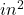Force applied on the piston(F) = 6.4 lb

Pressure on the piston (P) =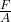⇒ P =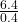⇒ P = 16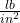This is the pressure inside the cylinder.

Let force applied on the brake pad =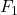Area of the brake pad (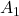)= 1.7Thus the pressure on the brake pad (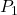) =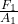When brake is applied on the vehicle the pressure on the piston is equal to pressure on the brake pad.

⇒ P =⇒ 16 == 16 ×Put the value ofwe get= 16 × 1.7= 27.2 lb

This the total force applied during braking.

The force applied on one wheel =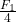=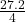= 6.8 lb

⇒ The force applied on one wheel during braking.